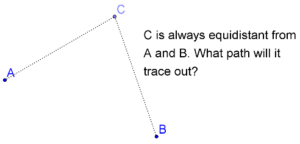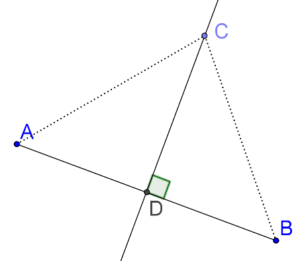# Perpendicular Bisectors

Go back to  'Triangles-Quadrilaterals'

Consider two fixed points A and B in the plane. Suppose that C is a moving point which moves in such a way so that it is always equidistant (at the same distance) from A and B. What will be the path traced out by C? In technical language, what will be the locus of C, given this constraint? Locus is a Latin word, and you can think of it as the path traced out by a point under a given set of conditions.The answer to this question is: the path traced out by C will be the perpendicular bisector of the segment joining A and B. In other words, if C lies anywhere on the perpendicular bisector of AB, it will necessarily be equidistant from A and B.

Let us see how. Consider the following figure, in which C is an arbitrary point on the perpendicular bisector of AB (which intersects AB at D):Compare ∆ACD and ∆BCD. We have:

1. AD = BD

2. CD = CD (common)

3. ∠ADC = ∠BDC = 90°

We see that ∆ACD ≡ ∆BCD by the SAS criterion. Thus, CA = CB, which means that C is equidistant from A and B.

To repeat this extremely important point once again: If a point C is equidistant from two fixed points A and B, then it must lie on the perpendicular bisector of A and B. Every point on the perpendicular bisector of AB will be equidistant from A and B.